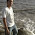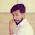» » Swap two numbers without using third variable

# Swap two numbers without using third variable

1. Java Interview Program to Swap two numbers without using third variable in java

1. package com.instaceofjava;
2.
3. public class SwapTwoNumbers {
4.
5. public static void main(String[] args) {
6.
7. int number1=20;
8. int number2=30;
9.
10. System.out.println("Before Swapping");
11. System.out.println("Value of number1 is :" + number1);
12. System.out.println("Value of number2 is :" +number2);
13.
14. number1=number1+number2;
15. number2=number1-number2;
16. number1=number1-number2;
17.
18. System.out.println("After Swapping");
19. System.out.println("Value of number1 is :" + number1);
20. System.out.println("Value of number2 is :" +number2);
21.
22. }
23. }
Output:

1. Before Swapping
2. Value of number1 is :20
3. Value of number2 is :30
4. After Swapping
5. Value of number1 is :30
6. Value of number2 is :20

#### 2. Java Program to Swap two numbers by using division and multiplication.

1. package com.instaceofjava;
2.
3. public class SwapTwoNumbers {
4.
5. public static void main(String[] args) {
6.
7. int number1=20;
8. int number2=30;
9.
10. System.out.println("Before Swapping");
11. System.out.println("Value of number1 is :" + number1);
12. System.out.println("Value of number2 is :" +number2);
13.
14. number1=number1*number2;
15. number2=number1/number2;
16. number1=number1/number2;
17.
18. System.out.println("After Swapping");
19. System.out.println("Value of number1 is :" + number1);
20. System.out.println("Value of number2 is :" +number2);
21.
22. }
23. }

Output:

1. Before Swapping
2. Value of number1 is :20
3. Value of number2 is :30
4. After Swapping
5. Value of number1 is :30
6. Value of number2 is :20

#### 3. Java Program to Swap two integers by using bit wise operators

1. package com.instaceofjava;
2.
3. public class SwapTwoNumbers {
4.
5. public static void main(String[] args) {
6.
7. int number1=2;
8. int number2=4;
9.
10. System.out.println("Before Swapping");
11. System.out.println("Value of number1 is :" + number1);
12. System.out.println("Value of number2 is :" +number2);
13.
14. number1=number1^number2;
15. number2=number1^number2;
16. number1=number1^number2;
17.
18. System.out.println("After Swapping");
19. System.out.println("Value of number1 is :" + number1);
20. System.out.println("Value of number2 is :" +number2);
21.
22. }
23. }
Output:

1. Before Swapping
2. Value of number1 is :2
3. Value of number2 is :4
4. After Swapping
5. Value of number1 is :4
6. Value of number2 is :2

#### Instance Of Java

We will help you in learning.Please leave your comments and suggestions in comment section. if you any doubts please use search box provided right side. Search there for answers thank you.

#### 3 comments for Swap two numbers without using third variable

1.1) and 2) doesn't work for all valid integers.
Take for 1) the following numbers: 1 and Integer.MAX_VALUE
Take for 2) the following numbers: any integer and 0
3) Could work

1.The first method is working fine with large values.
I have tested with Integer.MAX_VALUE-20 and Integer.MAX_VALUE using JRE 1.8.0

Calculation :
x = x + y
= 2147483627 + 2147483647
= -22

y = x - y
= -22 - 2147483647
= 2147483627 (don't know how JVM calculated this)

x = x - y
= -22 - 2147483627
= 2147483647 (don't know how JVM calculated this)

I am trying to figure out how JVM calculates it. If someone knows the calculation logic. Please explain.

2.you Still trying ??